# Setting the region¶

Many of the plotting functions take the `region` parameter, which sets the area that will be shown in the figure. This tutorial covers the different types of inputs that it can accept.

```import pygmt
```

## Coordinates¶

A string of coordinates can be passed to `region`, in the form of xmin/xmax/ymin/ymax.

```fig = pygmt.Figure()
fig.coast(
# Set the x-range from 10E to 20E and the y-range to 35N to 45N
region="10/20/35/45",
# Set projection to Mercator, and the figure size to 15 centimeters
projection="M15c",
# Set the color of the land to light gray
land="lightgray",
# Set the color of the water to white
water="white",
# Display the national borders and set the pen thickness to 0.5p
borders="1/0.5p",
# Display the shorelines and set the pen thickness to 0.5p
shorelines="1/0.5p",
# Set the frame to display annotations and gridlines
frame="ag",
)
fig.show()
```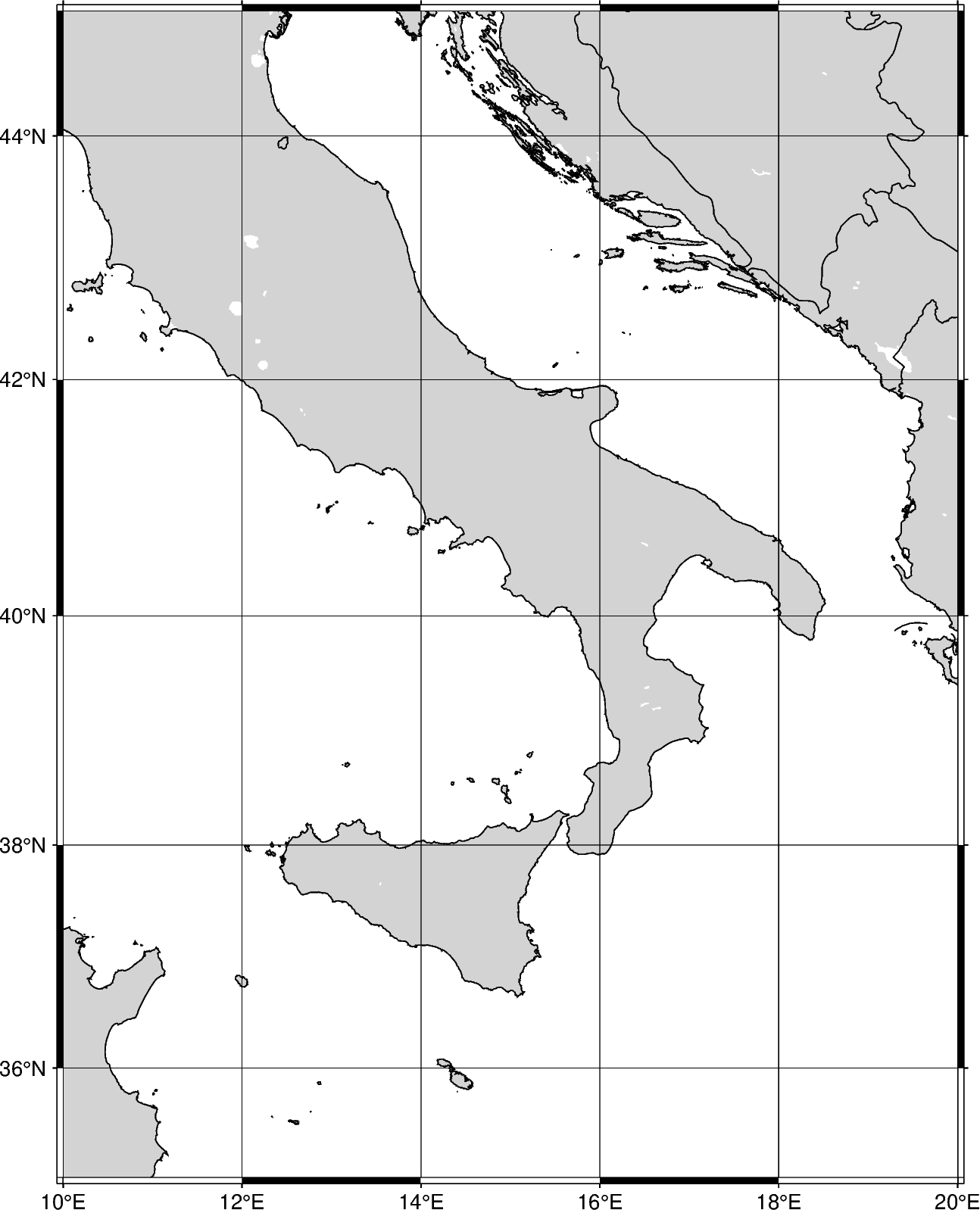Out:

```<IPython.core.display.Image object>
```

The coordinates can be passed to `region` as a list, in the form of [xmin,xmax,ymin,ymax].

```fig = pygmt.Figure()
fig.coast(
# Set the x-range from 10E to 20E and the y-range to 35N to 45N
region=[10, 20, 35, 45],
projection="M12c",
land="lightgray",
water="white",
borders="1/0.5p",
shorelines="1/0.5p",
frame="ag",
)
fig.show()
```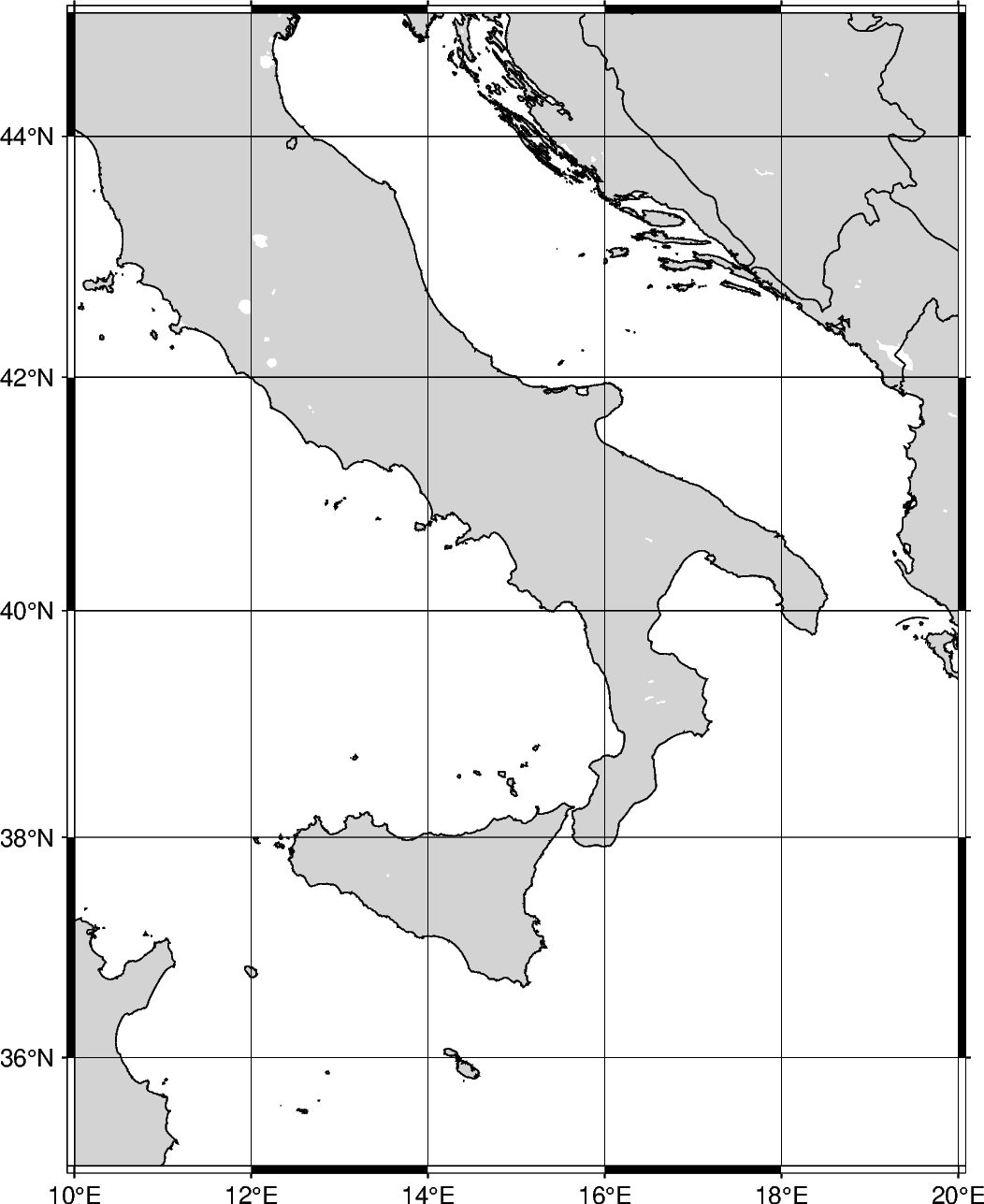Out:

```<IPython.core.display.Image object>
```

Instead of passing axes minima and maxima, the coordinates can be passed for the bottom-left and top-right corners. The string format takes the coordinates for the bottom-left and top-right coordinates. To specify corner coordinates, append +r at the end of the `region` string.

```fig = pygmt.Figure()
fig.coast(
# Set the bottom-left corner as 10E, 35N and the top-right corner as 20E, 45N
region="10/35/20/45+r",
projection="M12c",
land="lightgray",
water="white",
borders="1/0.5p",
shorelines="1/0.5p",
frame="ag",
)
fig.show()
```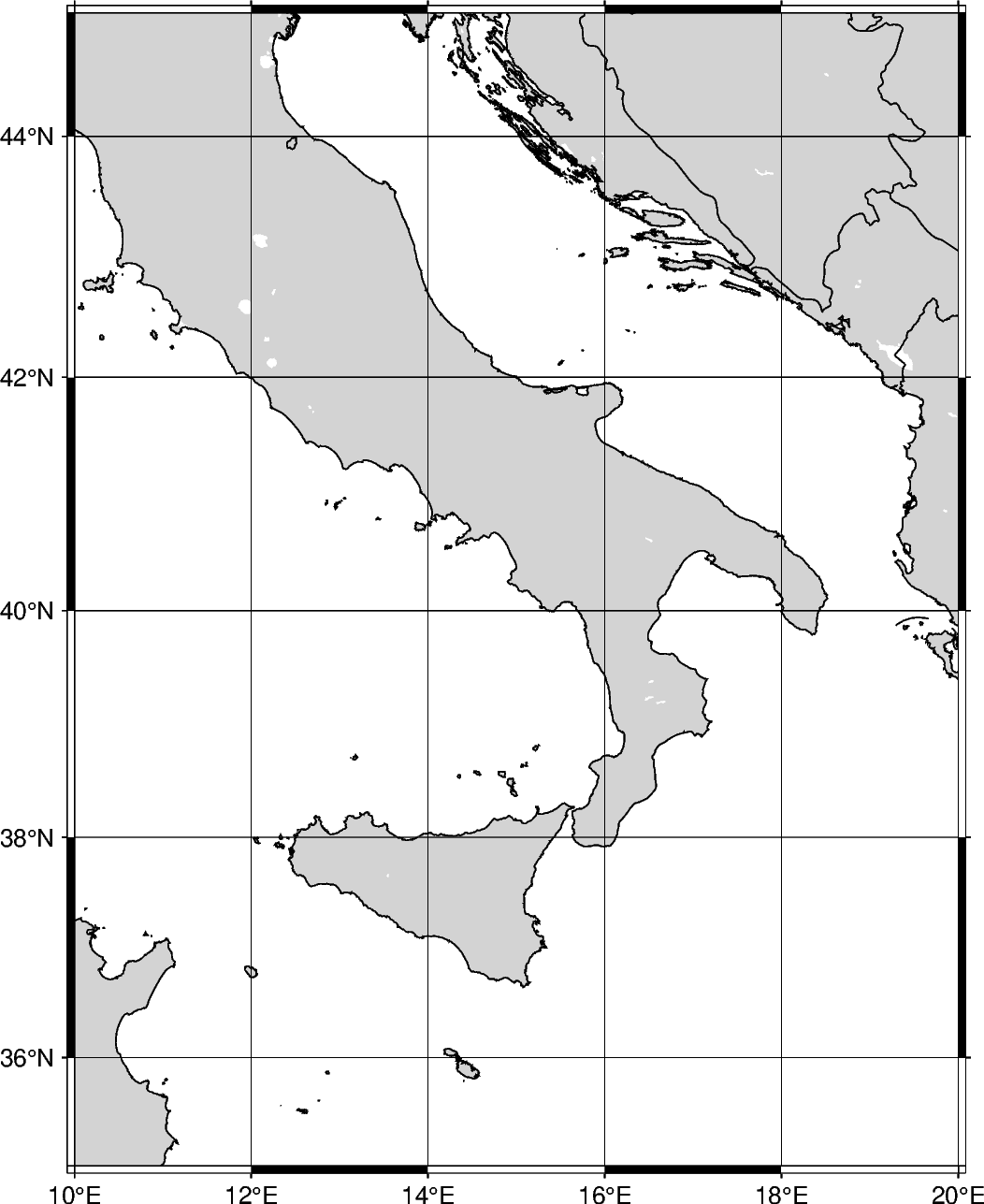Out:

```<IPython.core.display.Image object>
```

## Global regions¶

In addition to passing coordinates, the argument d can be passed to set the region to the entire globe. The range is 180W to 180E (-180, 180) and 90S to 90N (-90 to 90). With no parameters set for the projection, the figure defaults to be centered at the mid-point of both x- and y-axes. Using d, the figure is centered at (0, 0), or the intersection of the equator and prime meridian.

```fig = pygmt.Figure()
fig.coast(
region="d",
projection="Cyl_stere/12c",
land="darkgray",
water="white",
borders="1/0.5p",
shorelines="1/0.5p",
frame="ag",
)
fig.show()
```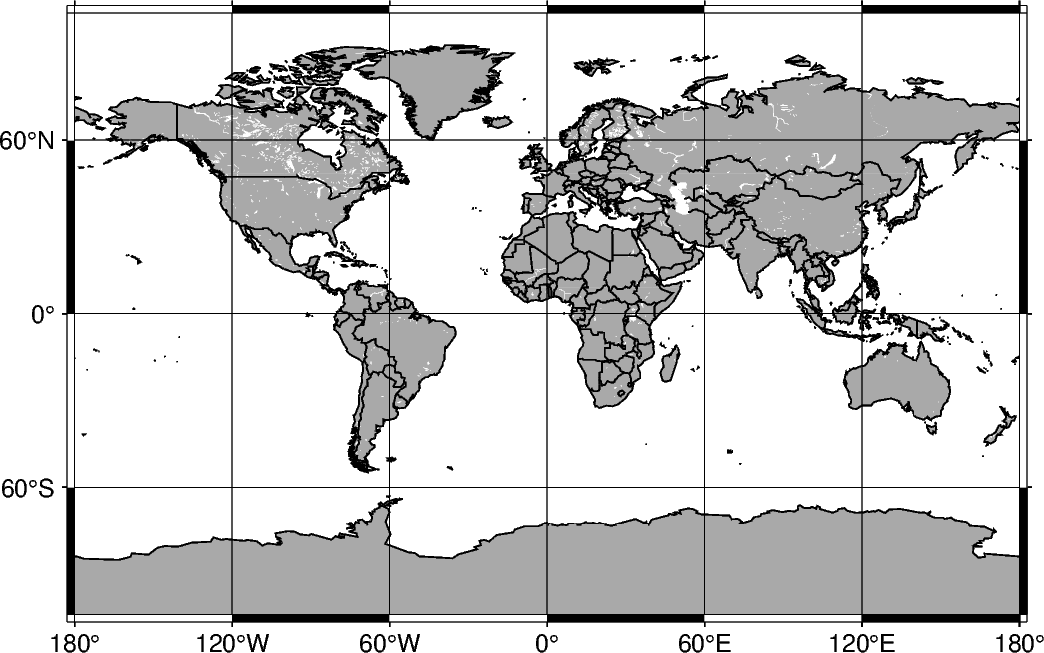Out:

```<IPython.core.display.Image object>
```

The argument g can be passed, which encompasses the entire globe. The range is 0E to 360E (0, 360) and 90S to 90N (-90 to 90). With no parameters set for the projection, the figure is centered at (180, 0), or the intersection of the equator and International Date Line.

```fig = pygmt.Figure()
fig.coast(
region="g",
projection="Cyl_stere/12c",
land="darkgray",
water="white",
borders="1/0.5p",
shorelines="1/0.5p",
frame="ag",
)
fig.show()
```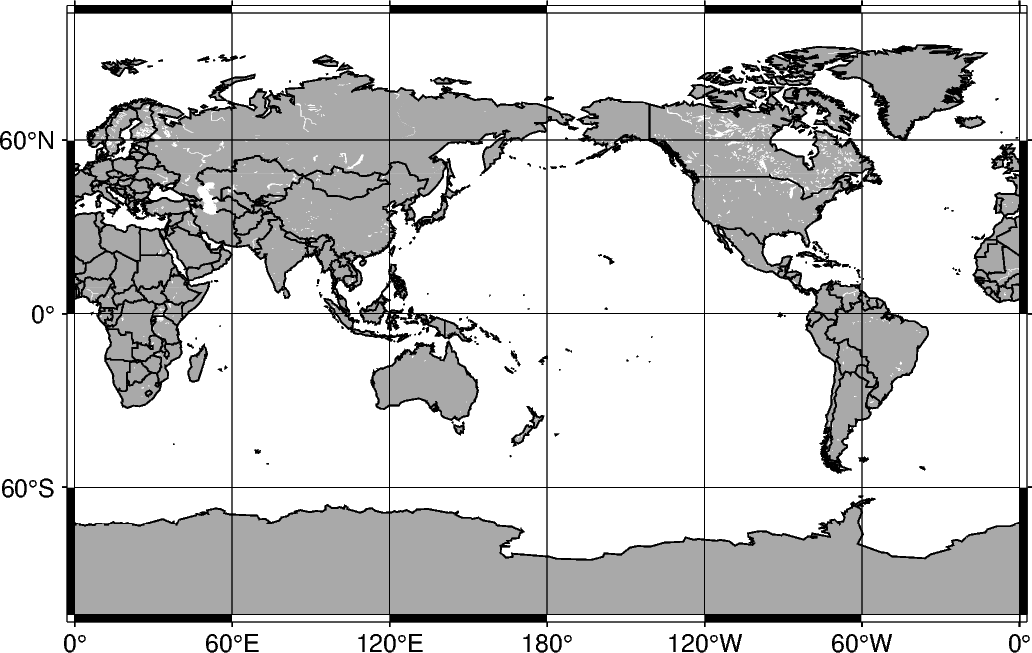Out:

```<IPython.core.display.Image object>
```

## ISO code¶

The `region` can be set to include a specific area specified by the two-character ISO 3166-1 alpha-2 convention (for further information: https://en.wikipedia.org/wiki/ISO_3166-1_alpha-2).

```fig = pygmt.Figure()
fig.coast(
# Set the figure region to encompass Japan with the ISO code "JP"
region="JP",
projection="M12c",
land="lightgray",
water="white",
borders="1/0.5p",
shorelines="1/0.5p",
frame="ag",
)
fig.show()
```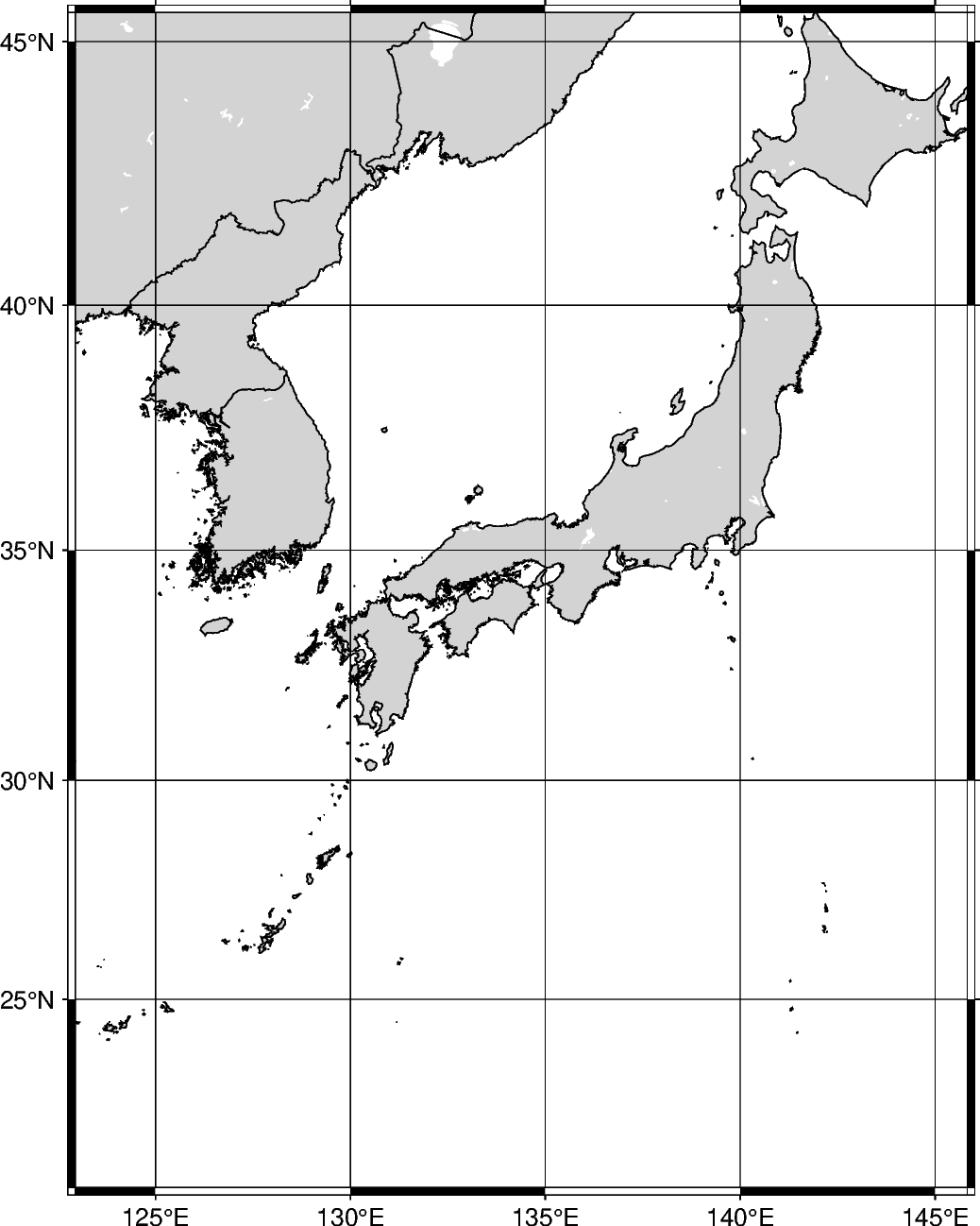Out:

```<IPython.core.display.Image object>
```

The area encompassed by the ISO code can be expanded by appending +rincrement to the ISO code. The increment unit is in degrees, and if only one value is added it expands the range of the region in all directions. Using +r expands the final region boundaries to be multiples of increment .

```fig = pygmt.Figure()
fig.coast(
# Expand the region boundaries to be multiples of 3 degrees in all directions
region="JP+r3",
projection="M12c",
land="lightgray",
water="white",
borders="1/0.5p",
shorelines="1/0.5p",
frame="ag",
)
fig.show()
```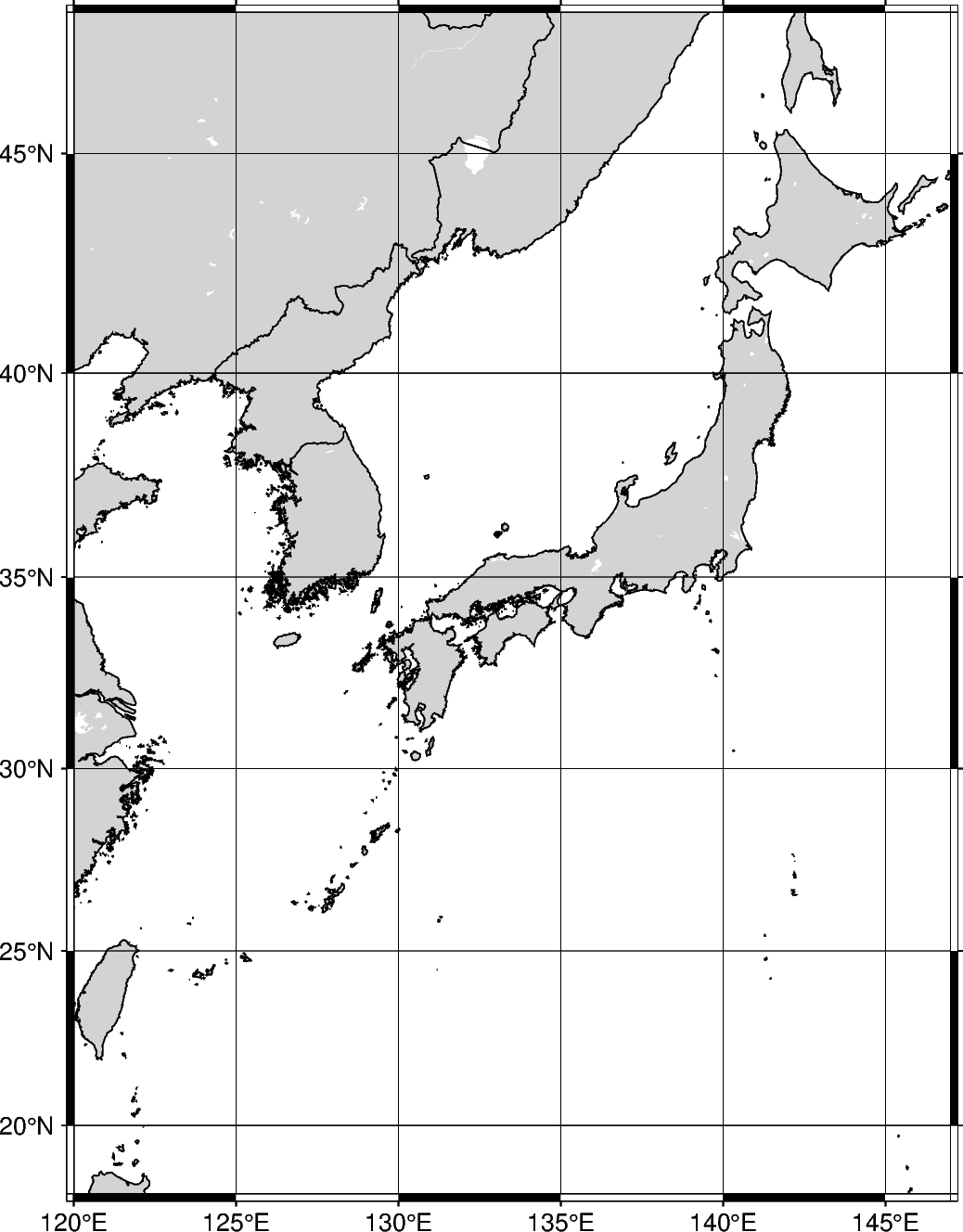Out:

```<IPython.core.display.Image object>
```

Instead of expanding the range of the plot uniformly in all directions, two values can be passed to expand differently on each axis. The format is xinc/yinc.

```fig = pygmt.Figure()
fig.coast(
# Expand the region boundaries to be multiples of 3 degrees on the x-axis
# and 5 degrees on the y-axis.
region="JP+r3/5",
projection="M12c",
land="lightgray",
water="white",
borders="1/0.5p",
shorelines="1/0.5p",
frame="ag",
)
fig.show()
```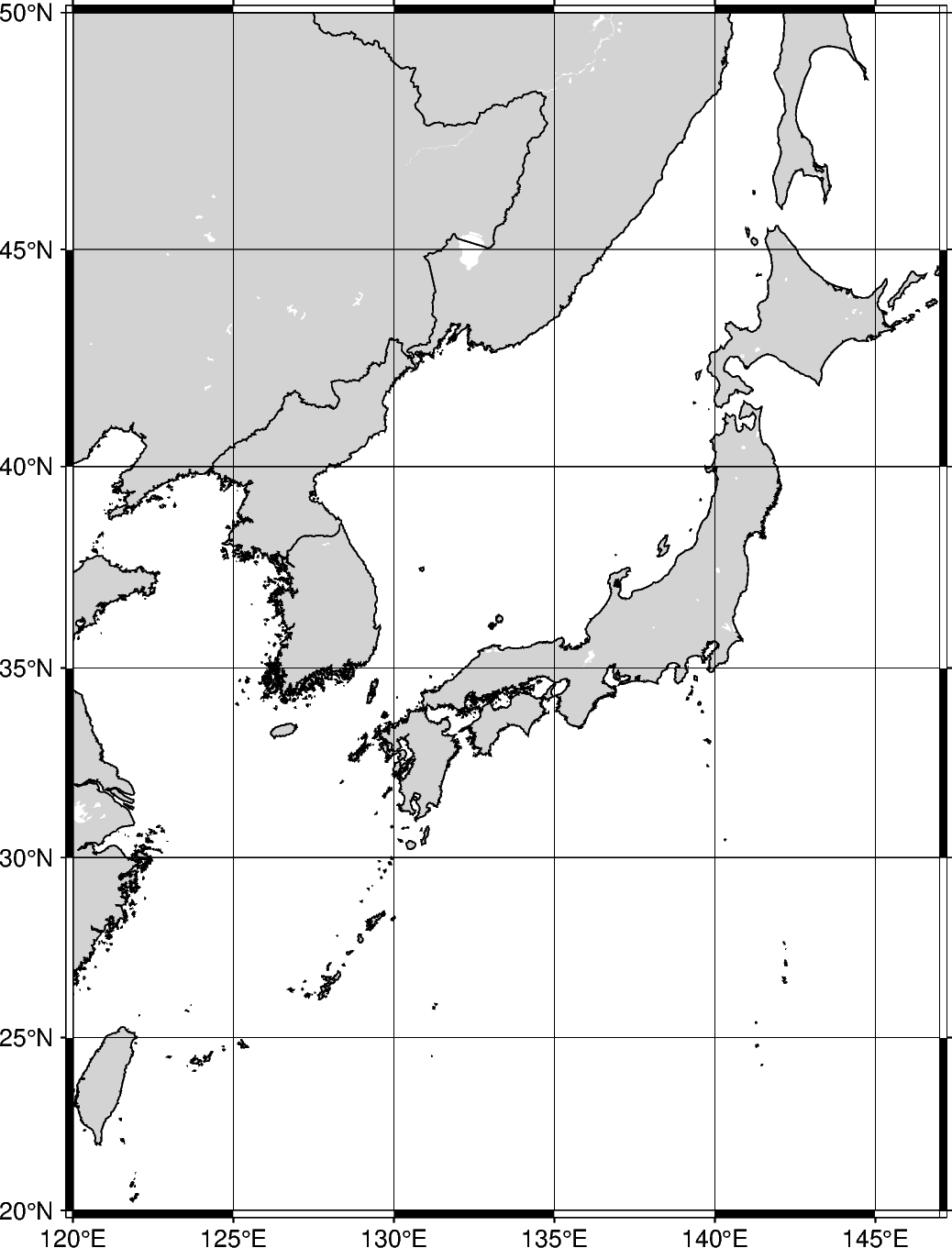Out:

```<IPython.core.display.Image object>
```

Instead of expanding the range of the plot uniformly in all directions, four values can be passed to expand differently in each direction. The format is winc/einc/sinc/ninc, which expands on the west, east, south, and north axes.

```fig = pygmt.Figure()
fig.coast(
# Expand the region boundaries to be multiples of 3 degrees to the west,
# 5 degrees to the east, 7 degrees to the south, and 9 degrees to the north.
region="JP+r3/5/7/9",
projection="M12c",
land="lightgray",
water="white",
borders="1/0.5p",
shorelines="1/0.5p",
frame="ag",
)
fig.show()
```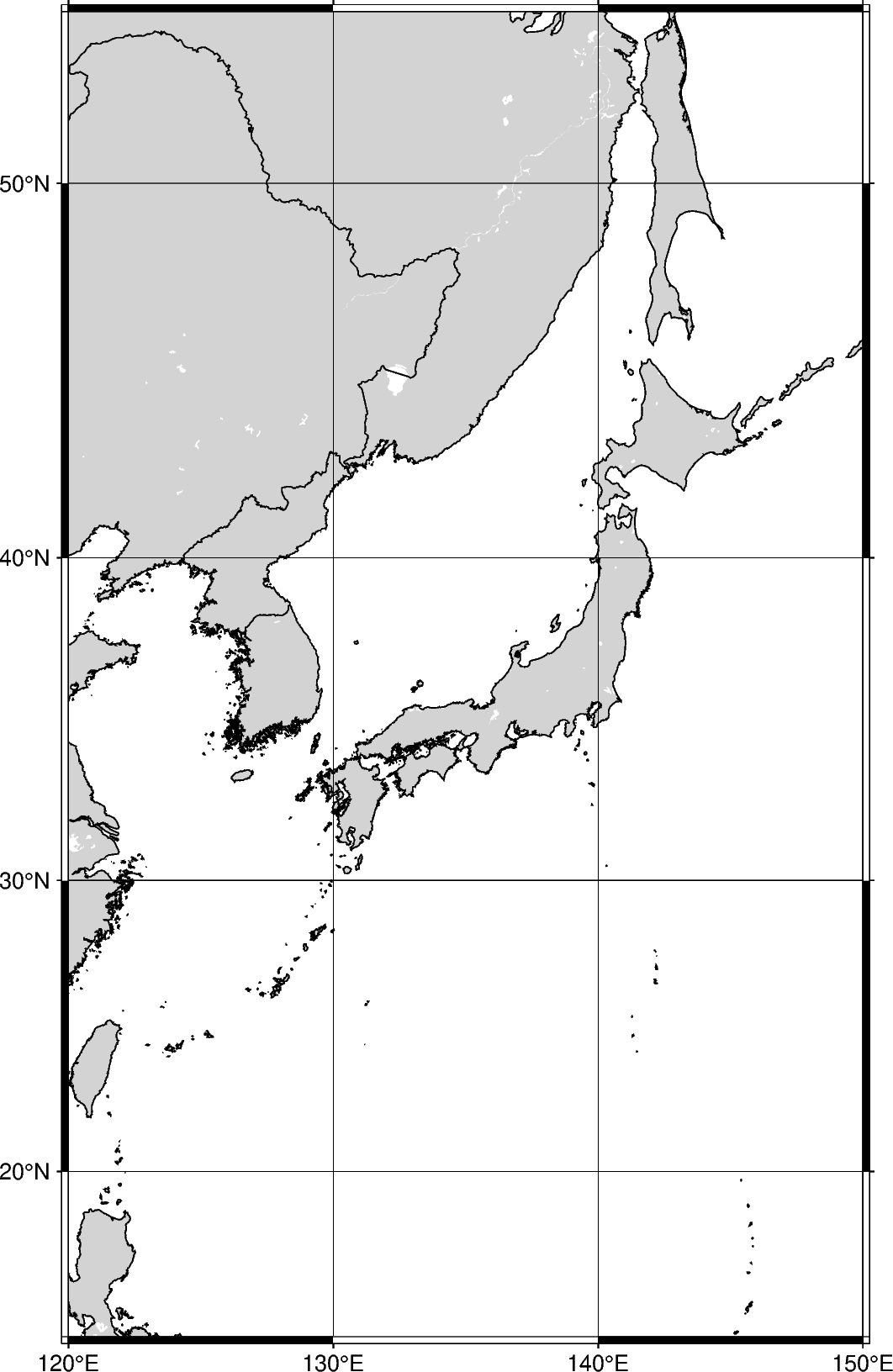Out:

```<IPython.core.display.Image object>
```

The `region` increment can be appended with +R, which adds the increment without rounding.

```fig = pygmt.Figure()
fig.coast(
# Expand the region setting outside the range of Japan by 3 degrees in all
# directions, without rounding to the nearest increment.
region="JP+R3",
projection="M12c",
land="lightgray",
water="white",
borders="1/0.5p",
shorelines="1/0.5p",
frame="ag",
)
fig.show()
```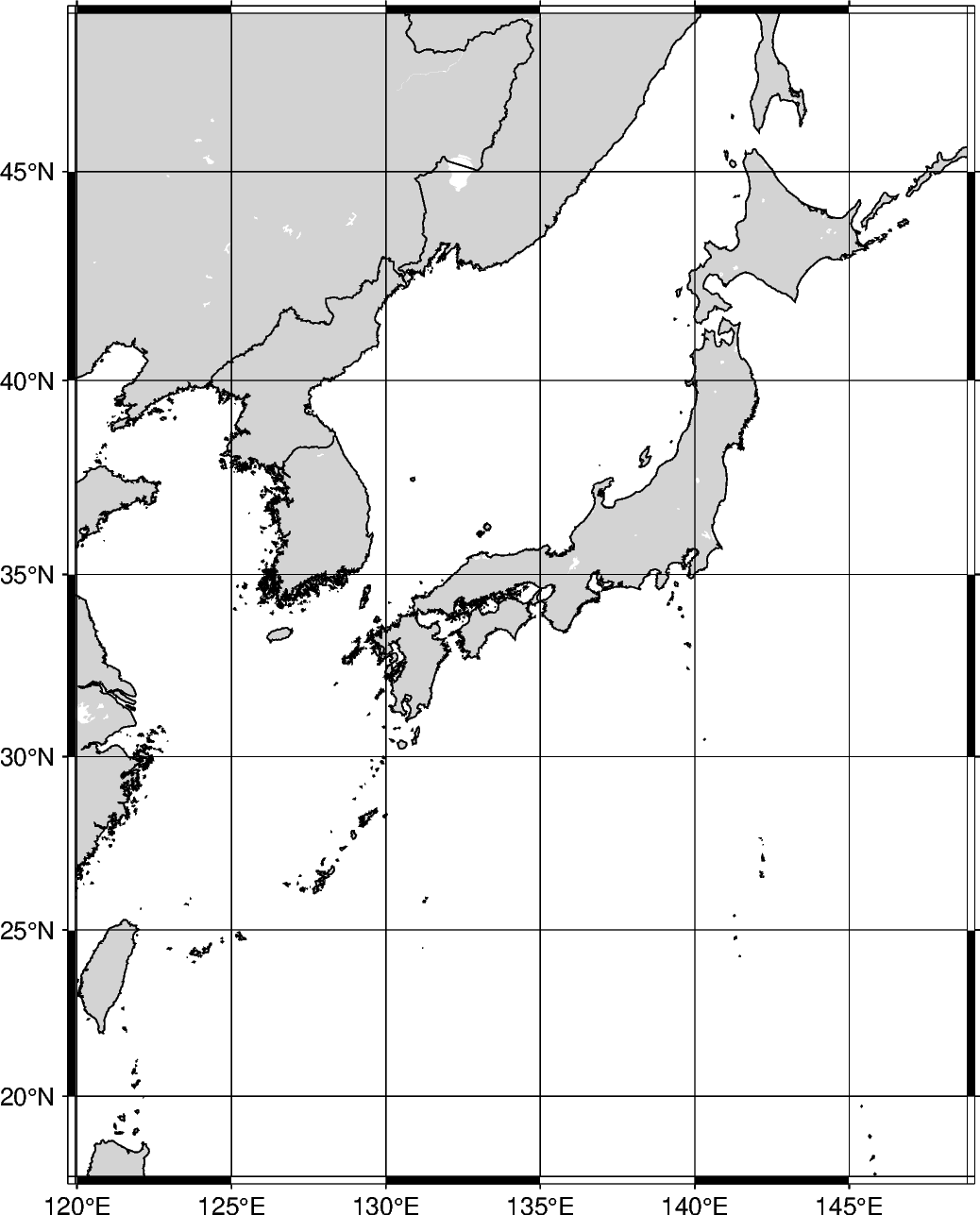Out:

```<IPython.core.display.Image object>
```

The `region` increment can be appended with +e, which is like +r and expands the final region boundaries to be multiples of increment. However, it ensures that the bounding box extends by at least 0.25 times the increment.

```fig = pygmt.Figure()
fig.coast(
# Expand the region boundaries to be multiples of 3 degrees in all directions
region="JP+e3",
projection="M12c",
land="lightgray",
water="white",
borders="1/0.5p",
shorelines="1/0.5p",
frame="ag",
)
fig.show()
```Out:

```<IPython.core.display.Image object>
```

Total running time of the script: ( 0 minutes 13.350 seconds)

Gallery generated by Sphinx-Gallery